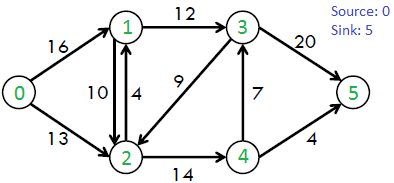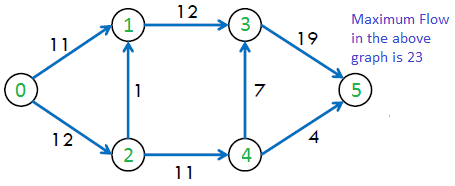Push Relabel Algorithm | Set 2 (Implementation)

• Difficulty Level : Hard
• Last Updated : 13 Jan, 2022

We strongly recommend to refer below article before moving on to this article.
Push Relabel Algorithm | Set 1 (Introduction and Illustration)

Problem Statement: Given a graph that represents a flow network where every edge has a capacity. Also given two vertices source ‘s’ and sink ‘t’ in the graph, find the maximum possible flow from s to t with following constraints:
a) Flow on an edge doesn’t exceed the given capacity of the edge.
b) Incoming flow is equal to outgoing flow for every vertex except s and t.

For example, consider the following graph from CLRS book.The maximum possible flow in the above graph is 23.Push-Relabel Algorithm
1) Initialize PreFlow : Initialize Flows and Heights

2) While it is possible to perform a Push() or Relablel() on a vertex
// Or while there is a vertex that has excess flow
Do Push() or Relabel()

// At this point all vertices have Excess Flow as 0 (Except source
// and sink)
3) Return flow.

Below are main operations performed in Push Relabel algorithm.

There are three main operations in Push-Relabel Algorithm

1. Initialize PreFlow() It initializes heights and flows of all vertices.

Preflow()
1) Initialize height and flow of every vertex as 0.
2) Initialize height of source vertex equal to total
number of vertices in graph.
3) Initialize flow of every edge as 0.
4) For all vertices adjacent to source s, flow and
excess flow is equal to capacity initially.

2. Push() is used to make the flow from a node that has excess flow. If a vertex has excess flow and there is an adjacent with a smaller height (in the residual graph), we push the flow from the vertex to the adjacent with a lower height. The amount of pushed flow through the pipe (edge) is equal to the minimum of excess flow and capacity of the edge.

3. Relabel() operation is used when a vertex has excess flow and none of its adjacents is at the lower height. We basically increase the height of the vertex so that we can perform push(). To increase height, we pick the minimum height adjacent (in residual graph, i.e., an adjacent to whom we can add flow) and add 1 to it.

Implementation

The following implementation uses the below structure for representing a flow network.

struct Vertex
{
int h;      // Height of node
int e_flow; // Excess Flow
}
struct Edge
{
int u, v; // Edge is from u to v
int flow; // Current flow
int capacity;
}
class Graph
{
Edge edge[];   // Array of edges
Vertex ver[];  // Array of vertices
}

The below code uses the given graph itself as a flow network and residual graph. We have not created a separate graph for the residual graph and have used the same graph for simplicity.